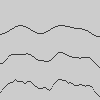# noise_detail()#

Adjusts the character and level of detail of Processing’s noise algorithm, produced by the noise() function.

## Examples#import numpy as np

def setup():
py5.noise_seed(42)
draw_noise_line(1, 0.25 * py5.height)
draw_noise_line(2, 0.50 * py5.height)
draw_noise_line(4, 0.75 * py5.height)

def draw_noise_line(octaves, y):
py5.noise_detail(octaves)
# use the noise method in a loop for clarity
for i in range(py5.width):
py5.point(i, y + 25 * py5.noise(i / 20))

# use the noise method with numpy arrays for efficiency
# xvals = np.arange(0, py5.width)
# yvals = y + 25 * py5.noise(xvals / 20)
# py5.points(np.stack([xvals, yvals]).T)import numpy as np

def setup():
py5.noise_seed(42)
py5.noise_detail(4, 0.5)
x, y = np.meshgrid(np.linspace(0, 5, py5.width), np.linspace(0, 5, py5.height))
new_pixels = py5.remap(py5.noise(x, y), 0, 1, 0, 255).astype(np.uint8)
py5.set_np_pixels(new_pixels, bands='L')


## Description#

Adjusts the character and level of detail of Processing’s noise algorithm, produced by the noise() function. Similar to harmonics in physics, Processing noise is computed over several octaves. Lower octaves contribute more to the output signal and as such define the overall intensity of the noise, whereas higher octaves create finer-grained details in the noise sequence.

By default, noise is computed over 4 octaves with each octave contributing exactly half than its predecessor, starting at 50% strength for the first octave. This falloff amount can be changed by adding an additional function parameter. For example, a falloff factor of 0.75 means each octave will now have 75% impact (25% less) of the previous lower octave. While any number between 0.0 and 1.0 is valid, note that values greater than 0.5 may result in noise() returning values greater than 1.0 or less than 0.0.

By changing these parameters, the signal created by the noise() function can be adapted to fit very specific needs and characteristics.

Underlying Processing method: noiseDetail

## Signatures#

noise_detail(
lod: int,  # number of octaves to be used by the noise
/,
) -> None

noise_detail(
lod: int,  # number of octaves to be used by the noise
falloff: float,  # falloff factor for each octave
/,
) -> None


Updated on March 06, 2023 02:49:26am UTC